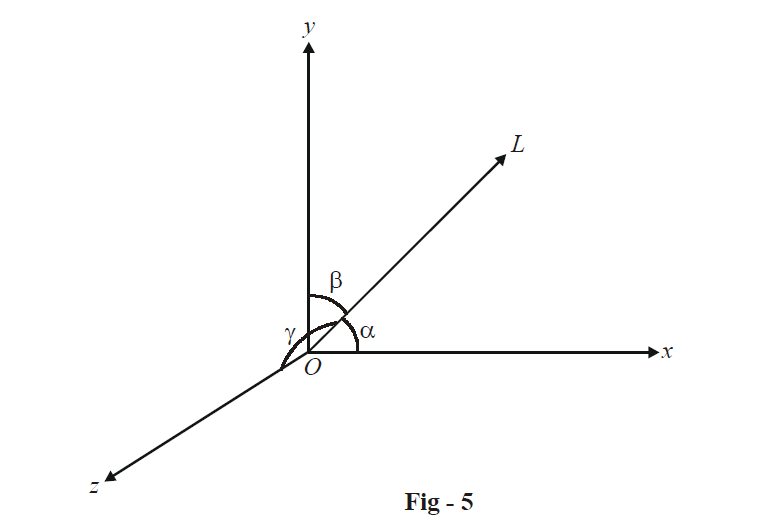# Direction Cosines and Ratios of Lines

## DIRECTION COSINES AND DIRECTION RATIOS

The direction cosines of a (directed) line are the cosines of the angles which the line makes with the positive directions of the coordinate axes.

Consider a line OL as shown, passing through the origin O. Let OL be inclined at angles  $$\alpha ,\,\beta ,\,\gamma$$   to the coordinate axes.Thus, the direction cosines are given by

$l = \cos \alpha ,\,\,\,m = \cos \beta ,\,\,\,\,n = \cos \gamma$

Note that for the line LO (i.e., the directed line segment in the direction opposite to OL), the direction cosines will be –l, –m, –n.

The direction cosines for a directed line Lnot passing through the origin are the same as the direction cosines of the directed line parallel to L and passing through the origin.

Note that for any point P lying on the line OL with direction cosines l, m, n such that OP = r, the coordinates of P will be

\begin{align}&\qquad\;\; x = lr,\,\,\,\,\,y = mr,\,\,\,\,\,z = nr \\ \\&\Rightarrow \quad p \equiv \left( {lr,\,mr,\,nr} \right) \\ \end{align}

Now, since

\begin{align} & \qquad \;\;\;\;\;OP = r \\\\ &\Rightarrow\quad \sqrt {{l^2}{r^2} + {m^2}{r^2} + {n^2}{r^2}} = r \\\\ &\Rightarrow\quad \boxed{{l^2} + {m^2} + {n^2} = 1} \\\end{align}

The direction cosines of any line will satisfy this relation.

The direction ratios are simply a set of three real numbers a, b, c proportional to l, m, n, i.e.

$\frac{l}{a} = \frac{m}{b} = \frac{n}{c}$

From this relation, we can write

\begin{align} &\qquad\;\; \frac{a}{l} = \frac{b}{m} = \frac{c}{n} = \pm \frac{{\sqrt {{a^2} + {b^2} + {c^2}} }}{{\sqrt {{l^2} + {m^2} + {n^2}} }} = \sqrt {{a^2} + {b^2} + {c^2}} \\ \\ &\Rightarrow \quad \boxed{l = \pm \frac{a}{{\sqrt {{a^2} + {b^2} + {c^2}} }},\,\,\,m = \pm \frac{b}{{\sqrt {{a^2} + {b^2} + {c^2}} }},\,\,\,n = \pm \frac{c}{{\sqrt {{a^2} + {b^2} + {c^2}} }}} \\ \end{align}

These relations tell us how to find the direction cosines from direction ratios.

Note that the direction cosines for any line must be unique. However, there are infinitely many sets of direction ratios since direction ratios are just a set of any three numbers proportional to the direction cosines.

Learn from the best math teachers and top your exams

• Live one on one classroom and doubt clearing
• Practice worksheets in and after class for conceptual clarity
• Personalized curriculum to keep up with school